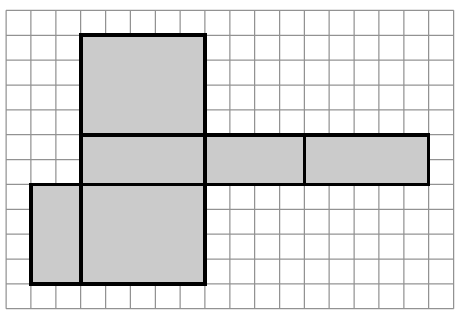### Home > CCG > Chapter 12 > Lesson 12.2.2 > Problem12-76

12-76.Find the volume and surface area of the box formed by the shaded net at right.

To find the surface area, find the area of each of the regions and sum them.

To find the volume, choose one area to be the base,
find the area and multiply it by the height.

$\text{Volume}=40\;\text{un}^3$